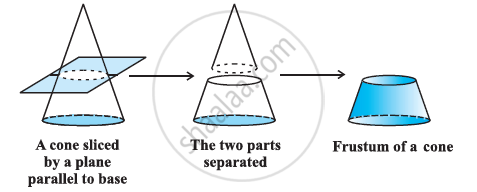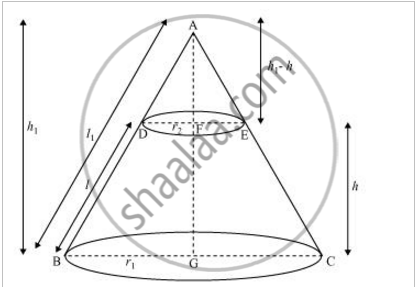# Frustum of a Cone

#### definition

A frustum may be formed from a right circular cone by cutting off the tip of the cone with a cut perpendicular to the height, forming a lower base and an upper base that are circular and parallel.#### notesIn ΔAFE and ΔAGC

ΔAFE ΔAGC (Angle-Angle rule)

"AF"/"AG"= "FE"/"GC"= "AE"/"AC"

(h_1-h)/(h_1)= r_2/r_1= (l_1-l)/l_1

(r_2)/(r_1)= (l_1-l)/(l_1)

r_2l_1= r_1(l_1-l)

r_2l_1= r_1l_1- r_1l

r_1l= r_1l_1- r_2l_1

r_1l= l_1 (r_1-r_2)

l_1= (r_1l)/ (r_1-r_2) ...........eq1

(h_1-h)/h_1= r_2/r_1= (l_1-l)/l_1

(h_1-h)/h_1= r_2/r_1

r_1(h_1-h)= h_1r_2

r_1h_1- r_1h= h_1r_2

r_1h_1- r_2h_1= r_1h

h_1(r_1-r_2)= r_1h

h_1= (r_1h)/(r_1-r_2) ............eq2

1) Curved surface area of frustum= Curved surface area of ABC- Curved surface area of ADE

= pi r_1l_1- pi r_2 (l_1-l)

= pi r_1l_1- pi r_2l_1 + pi r_2l

= pi l_1 (r_1- r_2) + pi r_2l

= pi r_1/(r_1-r_2) (r_1-r_2) +pi r_2l       (from eq1)

= pi r_1l + pi r_2l

Curved surface area of frustum = pi l(r_1+r_2)

2) Total surface area of frustum= Curved surface area of frustum + area of the circle above+ area of the circle below

Total surface area of frustum = pi l (r_1+r_2) + pi r_1^2+ pi r_2^2

3) Volume of frustum= Volume of cone ABC- Volume of cone ADE

= 1/3  pi r_1^2h_1- 1/3  pi r_2^2 (h_1-h)

=1/3   pi r_1^2h_1- 1/3   pi r_2^2h_1+ 1/3 pi r_2^2h

= 1/3   pi h_1 (r_1^2- r_2^2) +1/3   pi r_2^2h

= 1/3  pi (r_1h)/(r_1-r_2) (r_1^2-r_2^2)+ 1/3  pi r_2^2h     (from eq2)

= 1/3   pi (r_1h)/(r_1-r_2) (r_1-r_2) (r_1-r_2)+ 1/3 pi r_2^2h

= 1/3   pi r_1h (r_1+r_2)+ 1/3   pi r_2^2h

= 1/3 pi h [r_1 (r_1+r_2)+ r_2^2]

Volume of frustum= 1/3   pi h (r_1^2+ r_2^2+ r_1r_2)

Example- Hanumappa and his wife Gangamma are busy making jaggery out of sugarcane juice. They have processed the sugarcane juice to make the molasses, which is poured into moulds in the shape of a frustum of a cone having the diameters of its two circular faces as 30 cm and 35 cm and the vertical height of the mould is 14 cm. If each cm^3 of molasses has mass about 1.2 g, find the mass of the molasses that can be poured into each mould. (Take  pi= 22/7)

Solution : Since the mould is in the shape of a frustum of a cone, the quantity (volume) of molasses that can be poured into it = pi/3   h(r_1^2+ r_2^2+ r_1r_2)

where r_1 is the radius of the larger base and r_2 is the radius of the smaller base

=1/3 xx 22/7 xx 14 [(35/2)^2+ (30/2)^2= (35/2 xx 30/2)] cm^3

It is given that 1 cm^3 of molasses has mass 1.2g. So, the mass of the molasses that can be poured into each mould = (11641.7 xx 1.2) g

= 13970.04 g = 13.97 kg = 14 kg   (approx.)

If you would like to contribute notes or other learning material, please submit them using the button below.

#### Video Tutorials

We have provided more than 1 series of video tutorials for some topics to help you get a better understanding of the topic.

Series 1

Series 2

### Shaalaa.com

Surface Area and Volume part 15 (Frustum Concepts) [00:03:15]
S
0%